.yuimenubaritemlabel img{border:0;} .yuimenu {font:13px/1.22 arial,helvetica,clean,sans-serif;*font-size:small;*font:x-small;} #imvuminiheader { position:relative; }Get this badge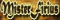My Videos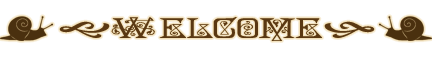Greetings and welcome to my home page. I work hard to bring you up to date quality products each month to satisfy your role play needs. Your continuous support helps keep my catalog alive. As a means to thank my frequent buyers, I have made this badge: Thank you and don't forget to review your favorite items. -Mister Firius
=•=•=•=•=•=•=•=•=•=•=•=•=•=•=•=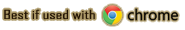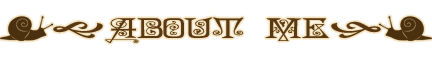=•=•=•=•=•=•=•=•=•=•=•=•=•=•=•==•=•=•=•=•=•=•=•=•=•=•=•=•=•=•=

 I started this secondary account when I first became a developer under Firiah (my main account), in order to have a male model for my products. That was before I knew male avatars were available for purchase in the catalog. The purpose of this account has varied over time, from secondary characters in different RP realms, to shop of RP and fandom items. Now it is my shop for mostly male items, with over hundreds of virtual products to choose from and with the compromise to keep adding more, as much as my real life allows me. One purpose it will never have is the deplorable practice of "catfish". I am a VERY straight woman behind these pixels, regardless of how drooling sexy my male avatar might look. Sorry girls, but you are wasting your time with your random seduction messages (yes, I have gotten a few... LOL).

=•=•=•=•=•=•=•=•=•=•=•=•=•=•=•=

=•=•=•=•=•=•=•=•=•=•=•=•=•=•=•=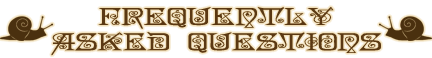=•=•=•=•=•=•=•=•=•=•=•=•=•=•=•=

Do you take requests?

Yes I do, but certain restrictions apply;

(Please notice that I charge for custom orders. For a list of prices, refer to the "How much do you charge for a request?" FAQ).

I require a minimum of two weeks for a quality product. If you need a last minute product, I recommend you ask someone else. Only exception to this rule is recoloring of already existing items in my catalog, which can take a minimum of 3 days (If the weather allows).

Requests I take:

• GA and AP outfits
• Furniture
• Recoloring of already existing items in my catalog
• Hairstyles and accessories, among other things
• Digital Pictures (DP)

Requests I do NOT take under any circumstances:

• UFI products (AKA Black Market items)
• Avatars and poses
• Skins, meshes and badges (I only work these at my discretion)
• Textures (I create my own and I don't want clones of my work)
• Replicas of someone else's textures (That's another form of theft)

NOTE: Taking these requests is strictly dependent of existing meshes (the shell) in the catalog. And ask BEFORE sending your credits, you need to make sure I can take your request first.

=•=•=•=•=•=•=•=•=•=•=•=•=•=•=•=

How much do you charge for a request?

For catalog customs (Items other people can buy), 5,000 credits per piece. (ie, if your outfit requires three pieces, then it is 5,000 for each piece for a total of 15,000).
The price covers submission and derivation fees that IMVU charges me, and I send it to you as soon as it passes peer review.

For exclusive request (Items no one else can have), 50,000 credits per piece. (ie, if your outfit requires three pieces, then it is 50,000 for each piece for a total of 150,000).
The price covers submission and derivation fees that IMVU charges me, the privilege of setting the item to DISPLAY ONLY so no one else can buy it, and I send it to you as soon as it passes peer review.

Digital pictures have a cost of 10,000 credits each. If animated, then the price is 15,000 credits.

*IMPORTANT* Ask BEFORE sending your credits, you need to make sure I can take your request first.

=•=•=•=•=•=•=•=•=•=•=•=•=•=•=•=

I am a submissive looking for a Master. Can you be him?

Your interest flatters me. However, I am afraid that's a big fat no! Sorry to burst your bubble, but I am not a man to start with.

I am not interested in slaves, subs or any sort of bondage and the drama that comes with it. I am just a role player, not a bondage "lifestyler". Therefore, I am not into "sexy" relationships outside of role play.

Please notice it is for me to choose my role play partners, not the other way around. And my real life stays out of IMVU.

=•=•=•=•=•=•=•=•=•=•=•=•=•=•=•=

Can you teach me how to create?

I'm afraid I can't. I am a very busy person and I don't have time to mentor anyone.

If you really interest learning how to create, IMVU provides plenty of tutorial resources from which you can take your sweet time to read and learn.

In some cases, they even provide step by step videos you can follow at your own pace.

=•=•=•=•=•=•=•=•=•=•=•=•=•=•=•=

Do you need a model for your products?

Thank you so much for your interest, but generally I am my own model for the product pages.

I also belong to several advertising groups that take care of the modeling for me, so I am well covered.

Perhaps you would like to join a model agency instead?

=•=•=•=•=•=•=•=•=•=•=•=•=•=•=•=

I tried to invite you, but you are in creator mode...

*Face Palms* How can I answer this without being disrespectful? Hmm.. Ok here I go:

Dear friend/customer, just like you, I also deserve respect. If I am in creator mode, that should be your hint that I might be busy deriving. I work a lot on deadlines and I do not appreciate interruptions when I am at it.

At times, I park at my public room to avoid a gazillion individual invites. You can go there if you truly want to see me, but be aware that response delays might occur if I am deriving.

I hope you understand my position on this and do not retort to uncalled insults because I am allegedly "ignoring" you.

=•=•=•=•=•=•=•=•=•=•=•=•=•=•=•=

Can you make me a texture for a product I am deriving?

Absolutely no. I spend hours editing images to create my own style, so I can make my own textures. I do not appreciate having clones of my work all over the shop. If I can come up with something creative, so can you. Practice is the key.

On that note, all of my products and textures are DMCA protected, so it is useless to try and steal from me.
Just saying...

=•=•=•=•=•=•=•=•=•=•=•=•=•=•=•=

Hang out with me now, or I will not support you anymore...

If that's how you feel, then so be it. But IMVU is not the only thing I do, even though it might seem that way at times. Just like you, I have a life outside of my computer that will ALWAYS be priority. If I miss an invite of yours, chances are I am multitasking to create products for your enjoyment or away from my computer, in an equally important task. I'm not ignoring you on purpose, keep this in mind before insulting me.

If it's a life threatening matter or a request, inbox me respectfully and you will get the same courtesy in return. Remember that Customer does NOT equal owner, even if you buy my entire catalog. I know my rights and my time is mine to spend as I will.

My friends will be expected to know better.

=•=•=•=•=•=•=•=•=•=•=•=•=•=•=•=

Can you gift me credits or products?

Please notice that Developer or Creator does not equal Philanthropist. Contrary to the massive belief, we do NOT swim in credits. And even if we did, however we spend them is our decision, not yours. Donation requests, regardless of the cause, are also out of the question.

Many of us creators are not fond of beggars. With this said, spare yourself the embarrassment of being sent to the nearest Walmart for a prepaid card. We work hard for our credits, so will you.

=•=•=•=•=•=•=•=•=•=•=•=•=•=•=•=

I am hosting a beauty pageant, can you donate credits to fund the prizes?

I am sorry but this is another form of begging. I cannot conceive anyone wanting to host such a big event with the mindset of spending the hard earned credits of people they don't know, to keep it going. I believe the host in question should be able to fund their own events.

When I became a creator, I had to purchase a few prepaid cards to submit my products to the catalog, instead of pestering people to fund me. I did my best to offer my customers a quality looking product so they felt like investing in my catalog. And I continue to improve my skills to keep them returning. Same way, if I do not see any benefit for me as a creator in your event/pageant/runway/etc. then I don't see why I should fund it. And offering to display my banner in the soap box of an IMVU group nobody follows, as an incentive for my credits, is no business for me at all. I can do that on my own for free. Call me unethical if you will, but that doesn't erase the fact that you are trying to pick my pocket.

If you need sponsors, offer me a win-win deal in which both get benefits, and I might give it a thought. Otherwise, spare me the speech of where in Walmart to find the prepaid cards, because that's where I send all the beggars.

=•=•=•=•=•=•=•=•=•=•=•=•=•=•=•=

Can I add you as a friend?

No offense, but I like to get to know people before I add them. I am not accepting random friend requests due to incidents that have escalated badly. Unknown friend requests without an inbox message will be ignored.

On that note, just because a friend, significant someone, or relative of yours is in my buddy list, doesn't mean you have the right to be there too.

Same way (in the case of those already in), if you are at odds with someone in my list, it doesn't mean I have to block them for you. Your enemies are yours, not mine, and I don't drop friends unless they mess with me directly. Trying to coax me into doing so will only result in your own blocking. I choose my friends, not the other way around.

=•=•=•=•=•=•=•=•=•=•=•=•=•=•=•=

My question is not listed here

If that's the case, feel free to inbox me and I shall respond as soon as time allows me.

Thanks for your understanding on these matters and be blessed.

-The human behind the avatar.THESE ARE FRESHLY FROM THE OVEN

More are added weekly, so stay tuned!

=•=•=•=•=•=•=•=•=•=•=•=•=•=•=•=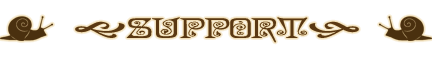(Click on the banner to visit my shop)=•=•=•=•=•=•=•=•=•=•=•=•=•=•=•=

Visit my other catalogs=•=•=•=•=•=•=•=•=•=•=•=•=•=•=•==•=•=•=•=•=•=•=•=•=•=•=•=•=•=•=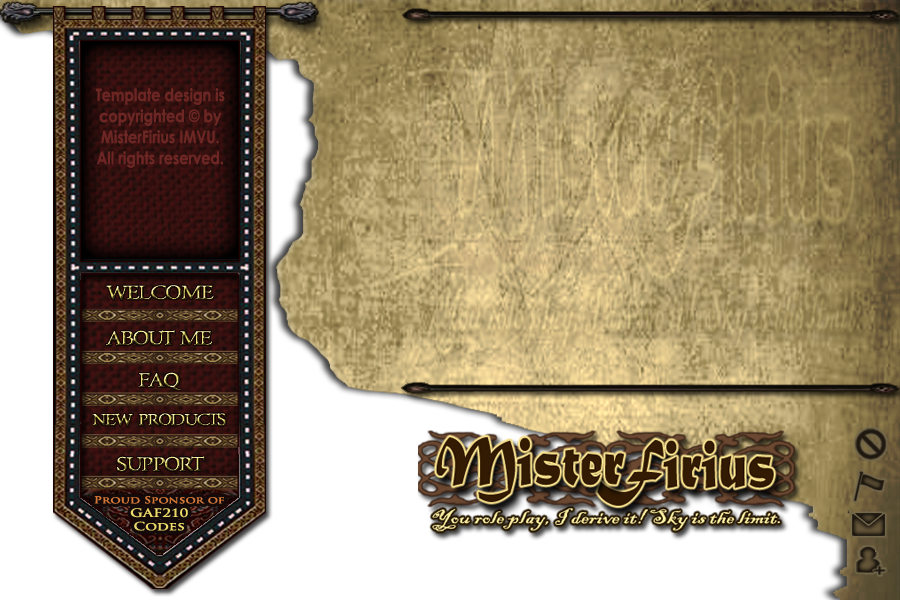© 2022 IMVU All rights reserved